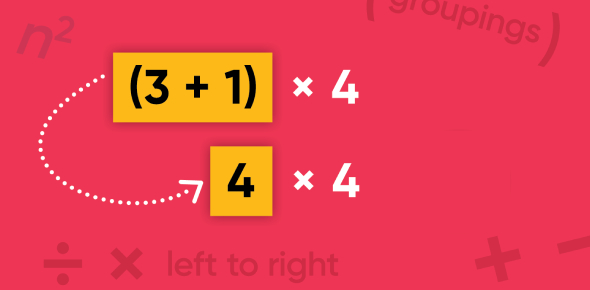# Can You Pass This Properties Of Addition And Multiplication Test?

10 Questions | Attempts: 1504SettingsAn Associative Property states that you can add or multiply regardless of how the numbers are grouped whereas, Commutative Property means the addition and multiplication of real numbers, integers, and rational numbers. This quiz has been created to test how well you are in solving and identifying the commutative and associative properties of addition and multiplication. So, let's try out the quiz. All the best!

• 1.
What number would make the number sentence true? 4x(?x6) = (4x3)x6
• A.

3

• 2.
What number will make the number sentence true? 48 x _____ = 0
• A.

0

• 3.
What property is illustrated by the fact below? 32 x 75 = 75 x 32
• A.

Commutative Property of Multiplication

• B.

Associative Property of Multiplication

• C.

• D.

• 4.
What number would make the number sentence tue? 44+(32+36)= (44+___)+36
• A.

32

• 5.
What number would make the number sentence true? 48 +____= 88 + 48
• A.

88

• 6.
Which property of addition is used below? 4 + 3 = 3 + 4
• A.

Associative property of multiplication

• B.

Commutative property of multiplication

• C.

• D.

• 7.
What number will make the number sentence true? 72 x ___ = 72
• A.

1

• 8.
Which property of addition is used below? (8+6)+3 = 8+(6+3)
• A.

Associative

• 9.
What number would make the number sentence true? 6x(5x9)= (6x__)x9
• A.

5

• 10.
( 4 + 5 ) + 7 = 4 + ( 5 + 7)
• A.

Associative property

• B.

Commutative property

• C.

Identity property

• D.

Distributive property

## Related TopicsBack to top
×

Wait!
Here's an interesting quiz for you.